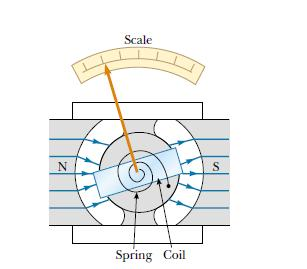×#### Thank you for registering.

One of our academic counsellors will contact you within 1 working day.

Click to Chat

1800-1023-196

+91-120-4616500

CART 0

• 0

MY CART (5)

Use Coupon: CART20 and get 20% off on all online Study Material

ITEM
DETAILS
MRP
DISCOUNT
FINAL PRICE
Total Price: Rs.

There are no items in this cart.
Continue Shopping• Complete JEE Main/Advanced Course and Test Series
• OFFERED PRICE: Rs. 15,900
• View Details

Solved Examples on Magnetostatics

Question 1:-

Suppose a cyclotron is operated at an oscillator frequency of 12 MHz and has a dee radius R = 53 cm.

(a) What is the magnitude of the magnetic field needed for deuterons to be accelerated in the cyclotron?

(b) What is the resulting kinetic energy of the deuterons?

Solution:-

(a) A deuteron has the same charge as a proton but approximately twice the mass (m = 3.34×10-27 kg). From equation qB = 2πmfosc,

B = 2πmfosc/q

= [(2π) (3.34×10-27kg)(12×106s-1)]/[1.6×10-19C]

= 1.57 T

Note that, to allow protons to be accelerated, B would have to be reduced by a factor of 2, assuming that the oscillator frequency remained fixed at 12 MHz.

(b) From equation r = mv/qB, the speed of the deuteron circulating with a radius equal to the dee radius R is given by

v = RqB/m

= [(0.53 m)(1.6×10-19C)(1.57 T)]/[3.34×10-27kg]

=3.99×107 m/s

This speed corresponds to a kinetic energy of

K = ½ mv2

= ½ (3.34×10-27kg)(3.99×107 m/s)2×(1MeV/1.60×10-13J)

= 16.6 MeV

From the above observation we conclude that, the resulting kinetic energy of the deuterons would be 16.6 MeV.Question 2:-

Analog voltmeters and ammeters work by measuring the torque exerted by a magnetic field on a current carrying coil. The reading is displayed by means of the deflection of a pointer over a scale. Below figure shows the basic galvanometer, on which both analog ammeters and analog voltmeters are based. The coil is 2.1 cm high and 1.2 cm wide; it has 250 turns and is mounted so that it can rotate about an axis (into the page) in a uniform radial magnetic field with B = 0.23 T. For any orientation of the coil, the net magnetic field through the coil is perpendicular to the normal vector of the coil. A spring provides a counter torque that balances the magnetic torque, so that a given steady current i in the coil results in a steady angular deflection ?. If a current of 100 µA produces an angular deflection of 28°, what must be the torsional constant κ of the spring, as used in equation  = -κ??Solution:-

Setting the magnetic torque [Equation,  = (NiA) B sinθ] equal to the spring torque and using absolute magnitudes yield

= NiA B sinθ = κ?,

in which ? is the angular deflection of the coil and pointer, and A (=2.52×10-4 m2) is the area encircled by the coil. Since the net magnetic field through the coil is always perpendicular to the normal vector of the coil, θ = 90° for any orientation of the pointer.

Solving the equation  = NiA B sinθ = κ? for κ, we find

κ = NiA B sinθ/?

= (250)(100×10-6 A) (2.52×10-4 m2)× [(0.23 T) (sin 90°)/28°]

= 5.2×10-8 N.m/degree

Many modern ammeters and voltmeters are of the digital, direct-reading type and operate in a way that does not involve a moving coil.

Question 3:-

(a) What is the magnetic dipole moment of the coil of Question 2, assuming that it carries a current of 100 µA?

(b) The magnetic dipole moment of the galvanometer coil is lined up with an external magnetic field whose strength is 0.85 T. How much work would be required to turn the coil end for end?

(c) What is the magnitude of the maximum torque  that the external field B can exert on the magnetic dipole moment?

Solution:-

(a) The magnitude of the magnetic dipole moment of the coil, whose area A is 2.52×10-4 m2, is

μ = NiA

= (250) (100×10-6A) (2.52×10-4 m2)

= 6.3×10-6 A. m2

= 6.3×10-6 J/T

Therefore, the magnetic dipole moment of the coil would be 6.3×10-6 J/T. You can show that these two sets of units are identical.

(b) The required work is equal to the increase in potential energy; that is, from equation ΔU = 2µB,

W = ΔU

= 2µB

= 2(6.3×10-6 J/T) (0.85 T)

= 10.7×10-6 J

≈ 11 µJ

From the above observation we conclude that, the work required to turn the coil end for end would be11 µJ.

(c) From equation =μB sinθ, the maximum torque occurs when the magnitude of sinθ is 1. Thus, we have

=μB sinθ

= (6.3×10-6 J/T) (0.85 T)(1)

=  5.4×10-6 N.m

Thus the maximum torque  that the external field B can exert on the magnetic dipole moment would be 5.4×10-6 N.m.### Course Features

• 728 Video Lectures
• Revision Notes
• Previous Year Papers
• Mind Map
• Study Planner
• NCERT Solutions
• Discussion Forum
• Test paper with Video Solution